Economics

# Assignment on Cost of Capital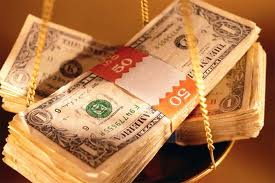COST OF CAPITAL

It is vitally important that a firm knows how much it pays for the funds used to purchase assets. The average return required by the firm’s investors determines how much must be paid to attract funds. The firm’s required rate of return is its average cost of funds, which more commonly is termed the cost of capital. The cost of capital is an important element, as basic input information in capital investment decision. It also referred to as cut-off-rate, target rate, hurdle rate, minimum required rate of return, standard return and so on.

1. Definition:

Conceptually the cost of Capital may be defines as the minimum rate of return that a firm must earn on its investment for the market value of the firm to remain unchanged.

In operational terms, the cost of capital is a discount rate that is used in determining the present value of future cash flows.

In other words, the cost of capital is the firm’s required rate of return, r. For example if investors provide funds to a firm for an average cost of 15 percent, wealth will decrease if the funds are used to generate returms less than 15 percent, wealth will not change if exactly 15 percent if earned, and wealth will increase if the firm generates return greature than 15 percent

The cost of capital (k) consist of the following three components

rj =The riskless cost of the particular type of financing;

Cost of Capital, k= rj+ b+f

2. IMPORTANCE OF COST OF CAPITAL

The concept of cost of capital is a very important concept in financial management decision making. The concept is however, a recent development and has relevance in almost every financial decision making but prior to that development, the problem was ignored or by-passed.

The progressive management always takes notice of the cost of capital while taking a financial decision. The concept is quite relevant in the following managerial decisions.

• Capital Budgeting Decision. Cost of capital may be used as the measuring road for adopting an investment proposal. The firm, naturally, will choose the project which gives a satisfactory return on investment which would in no case be less than the cost of capital incurred for its financing. In various methods of capital budgeting, cost of capital is the key factor in deciding the project out of various proposals pending before the management. It measures the financial performance and determines the acceptability of all investment opportunities.
• Designing the Corporate Financial Structure. The cost of capital is significant in designing the firm’s capital structure. The cost of capital is influenced by the chances in capital structure. A capable financial executive always keeps an eye on capital market fluctuations and tries to achieve the sound and economical capital structure for the firm. He may try to substitute the various methods of finance in an attempt to minimize the cost of capital so as to increase the market price and the earning per share.
• Deciding about the Method of Financing. A capable financial executive must have knowledge of the fluctuations in the capital market and should analyse the rate of interest on loans and normal dividend rates in the market from time to time. Whenever company requires additional finance, he may have a better choice of the source of finance which bears the minimum cost of capital. Although cost of capital is an important factor in such decisions, but equally important are the considerations of relating control and of avoiding risk.
• Performance of Top Management. The cost of capital can be used to evaluate the financial performance of the top executives. Evaluation of the financial performance will involve a comparison of actual profitabilities of the projects and taken with the projected overall cost of capital and an appraisal of the actual cost incurred in raising the required funds.
•  Other Areas. The concept of cost of capital is also important in many others areas of decision making, such as dividend decisions, working capital policy etc.

3. Explicit and Implicit Cost: The cost of capital can be either explicit or implicit.

Explicit cost: The rate of return of the cash flows of the financing opportunity. In other word it is the internal rate of return that the firm pays to procure financing.

Implicit Cost (opportunity cost): The rate of return associated with the best investment opportunity for the firm and its shareholders that would be foregone, if the projects presently under consideration by the firm were accepted.

4. FACTORS AFFECTING THE COST OF CAPITAL

Controllable Factors Affecting the Cost of Capital

These are the factors affecting cost of capital that the company has control over:

Capital Structure Policy

A firm has control over its capital structure, targeting an optimal capital structure. As more debt is issued, the cost of debt increases, and as more equity is issued, the cost of equity increases.

Dividend Policy

The firm has control over its payout ratio; the breakpoint of MCC schedule can be changed. For example, as the payout ratio of the company increases the breakpoint between lower-cost internally generated equity and newly issued equity is lowered.

Investment Policy

It is assumed that, when making investment decisions, the company is making investments with similar degrees of risk. If a company changes its investment policy relative to its risk, both the cost of debt and cost of equity change.

Uncontrollable Factors Affecting the Cost of Capital

These are the factors affecting cost of capital that the company has no control over:

Level of Interest Rates

The level of interest rates will affect the cost of debt and, potentially, the cost of equity. For example, when interest rates increase the cost of debt increases, which increases the cost of capital.

Tax Rates

Tax rates affect the after-tax cost of debt. As tax rates increase, the cost of debt decreases, decreasing the cost of capital.

5. MEASUREMENT OF SPECIFIC COSTS

The computation of the cost of capital, therefore involves two steps

• The computation of the different elements of the cost in terms of the different sources of finance (specific costs)
• The calculation of the overall cost by combining the specific costs in to composite cost.

In calculating the cost of capital, therefore, the focus is on long-term funds. In other words, the specific costs have to be calculated for (i) long-term debt (ii) preference Share (iii) equity capital (iv) retained earnings

5.1 COST OF DEBT:

The cost of funds raised through dept in the form of debentures or loan from financial institution called cost of dept.

The cost of debt is relatively simple to calculate, as it is composed of the rate of interest paid. In practice, the interest-rate paid by the company can be modeled as the risk-free rate plus a risk component risk premium, which itself incorporates a probable rate of default (and amount of recovery given default). For companies with similar risk or credit rating, the interest rate is largely exoginous (not linked to the company’s activities).

Cost of perpetual Debt: It is the rate of return which the lenders expect. The dept carries a certain rate of interest.

Formula

i.  ki    =

ii.  k=

Here,

ki = Before tax cost of dept

kd = Tax adjusted cost of dept

I = Annual interest payment

SV= Sales proceeds of the bond/ debenture

t = Tax rate

Problem 1: A company has 15% perpetual debt of tk. 100000. The tax rate is 35%. Determine the cost of capital (before tax as well as after tax) assuming the debt is issued at (i) par          (ii) 10% discount, and (iii) 10% premium.

Solution:

(i) Debt issued at par

Before- tax cost, k =

After-tax cost, k = k  (1-t) = 15 %( 1-0.35) = 9.75%

(ii) Issued at discount

Before- tax cost, k  =

After-tax cost, k = k  (1-t) = 16.7% (1-0.35) =10.85%

(ii) Issued at discount

Before- tax cost, k  =

After-tax cost, k = k  (1-t) = 13.6% (1-0.35) =8.84%

Problem 2: A company issues a new 15% debenture of tk. 1000 face value to be redeemed after 10 years. The debenture is expected to be sold at 5 percent discount. It will also involve flotation costs of 2.5% of face value. The company’s tax rate is 35%. What would the cost of debt be? Illustrate the computations using (i) trial and error approach and (ii) shortcut method.

Solution

(i) Trial and Error/ Long Approach

Cash flow pattern of the debenture would be as follows:

 Years Cash Flow 0 + Tk. 925 (tk.1000- tk.75, that is par value less flotation cost less discount) 1-10 – Tk. 150 (Interest outgo) 10 – Tk. 1000 ( repayment of principal at maturity

We are to determine the value of k in the following equation:

Tk.925 =

The value of k for this equation would be the cost of debt. The value of k can be obtained, as in the case of IRR by trial and error.

Determination of PV at 10% and 11% rates of interest

 Years Cash outflows PV factor at Total PV at 10% 11% 10% 11% 1-10 Tk. 97.5 6.145 5.889 Tk. 599.14 Tk.574.18 10 Tk. 1000 0.386 .352 Tk. 386.00 Tk. 352.00 Total =    Tk. 984.14       Tk. 926.18

The value of kwould be 11%.

(ii) Shortcut Method: The formula of approximating the effecting cost of debt can, as a shortcut, be showing the following equation: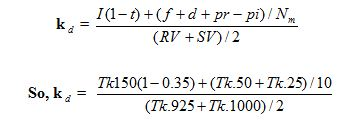5.2 COST OF PREFERENCE SHARES

The return required by the holders of preference shares.

The preference shares are usually cumulative which means that preference dividend will get accumulated till it is paid. There are two type of preference share: i. irredeemable (perpetual Security) ii. RedeemableProblem 1:  A company issues 14 % irredeemable preference shares of the face value of tk. 100 each. Flotation costs are estimated at 5% of the expected sale price.           (a) What is the coxt of preference shares (kp), if preference shares are issued at (i) par value (ii) 10 percent premium and (iii) 5 percent discount? (b) Also, compute kp in these solutions assuming 10 percent dividend tax.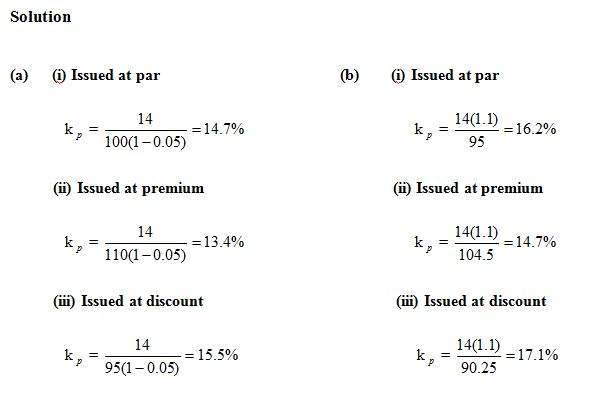kp = Cost of preference capital

D= Constant annual dividend payment

P0 = Expected sales price of preference shares

f    = Flotation costs as a percentage of sales price

Dt = Tax on preference dividend

Problem 1:  A company issues 14 % irredeemable preference shares of the face value of tk. 100 each. Flotation costs are estimated at 5% of the expected sale price.           (a) What is the coxt of preference shares (kp), if preference shares are issued at (i) par value (ii) 10 percent premium and (iii) 5 percent discount? (b) Also, compute kp in these solutions assuming 10 percent dividend tax.

Problem 2: ABC ltd has issued 14 percent preference shares of the face value of tk. 100 each to be redeemed after 10 years. Flotation cost is expected to be 5 percent. Determine the cost of preference shares.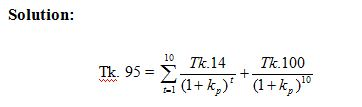The value of k is likely to be between 14 and 15 percent as the rate of dividend is 14 percent.

Determination of PV at 14 percent and 15 percent

So, cost of preference shares k = 15 percent

5.3 COST OF EQUITY CAPITAL (ke)

May be defined as the minimum rate of return that a firm must earn on the equity financed portion of an investment project in order to leave unchanged the market price of the share.

Dividend approach of Cost of Equity Capital (ke) may be defined as the discount rate that equates the present value of all expected future dividends per share with the net proceeds of the sale ( or the current market price) of a share.

The cost of equity is more challenging to calculate as equity does not pay a set return to its investors. Similar to the cost of debt, the cost of equity is broadly defined as the risk-weighted projected return required by investors, where the return is largely unknown. The cost of equity is therefore inferred by comparing the investment to other investments (comparable) with similar risk profiles to determine the “market” cost of equity.

The measurement of the above required rate of return is the measurement of the cost of equity capital. There are two possible approaches that can be employed to calculate the cost of equity capital:

(i) Dividend Approach (ii) Capital Asset Pricing Model Approach.

5.3.1 Dividend Approach

According to this approach, the cost of capital is calculated on the basis of required rate of return in terms of the future dividends to be paid on the shares. The cost of equity capital, ke, is accordingly, defined as the discount rate that equates the present value of all expected future dividens per share with the net proceeds of the sale of a share.

The two elements of the calculation of ke on the basisof the dividend approach are (i) net proceeds from the sale of a share/current market price of a share, and (ii) dividends and capital gains expected on the share.

The cost of equity capital can be measured with the following equations:

a) When dividends are expected to grow at a uniform rate perpetually:

k =

Here,

ke = The cost of equity capital

D1 = Expected Dividend per share

P0 = Net proceeds per share/ current market price

g= growth in expected dividends

Problem: Suppose that dividend per share is expected to be tk. 1 per share next year and is expected to grow at 6 percent per year perpetually. Determine the cost of equity capital, assuming the market price per share is tk. 25.

Solution

This is a case of expected dividends. The ke can be calculated by using the equation       k =, Thus k = = 10 percent.

The dividend approach can be used to determine the expected market value of a share in different years. The expected value of a share of the hypothetical firm in the above problem at the end of years 1 and 2 would be as follows:

(i)                 Price at the end of the first year (p) === tk.26.50.

(ii)               Price at the end of the first year (p) === tk.28

5.3.2 Capital Asset Pricing Model Approach (CAPM)

CAPM, which was developed in the mid 1960’s, explains the behaviour of security prices and provides a mechanism whereby investors could assess the impact of proposed security investment on their overall portfolio risk and return.

The Capital Asset Pricing Model uses various assumptions about markets and investor behavior to give a set of equilibrium conditions that allow us to predict the return of an asset for its level of systematic (or nondiversifiable) risk.

The basic assumption of CAPM is related to a) the efficiency of the security markets b) investors preference.

The efficient market assumption implies that

• All investors have common expectations regarding the expected returns, variances and correlation of returns among all securities.
• All investors have the same information about securities
• There are no restriction on investment
• There are no taxes
• There are no transaction costs
• No single investor can affect market price significantly

The implication of investors’ preference assumption is that all investors prefer the security that provides the highest return for a given level of risk or the lowest amount of risk for a given level of return, that is, the investors are risk averse.

Types of risk

• Diversifiable/ unsystematic: Also known as “specific risk,” this risk is specific to individual stocks and can be diversified away as the investor increases the number
• of stocks in his or her portfolio. In more technical terms, it represents the component of a stock’s return that is not correlated with general market moves.
• Non diversifiable / systematic: These are market risks that cannot be diversified away. Interest rates, recessions and wars are examples of systematic risks.

The CAPM uses a measure of systematic risk that can be compared with other assets in the market. Using this measure of risk can theoretically allow investors to improve their portfolios and managers to find their required rate of return. According to CAPM, the non-diversifiable risk of an asset/investment is assessed in terms of the beta coefficient. Beta is a measure of the volatility of a security’s return relative to he returns of a broad-based market portfolio. Beta coefficient is the overall risk in investing in a large market. A company’s beta coefficient is that company’s risk compared to the risk of the overall market. Beta coefficient of 1 implies that the risk of the specified security is equal to the market; the interpretation of zero coefficient is that there is no market related risk to the investment. If the company has a beta coefficient of 3.0, then it is said to be 3 times more risky than the overall market.

The Formula:

k = R + b (k-R)

Here,

ke = The cost of equity capital

Rf = the rate of return required on a risk-free asset

/security / investment

KM = The required rate of return on the market portfolio of assets that can be viewed as the average rate of return on all assets.

b= the beta coefficient

Problem: The Hypothetical Ltd wishes to calculate its cost of equity capital using Capital Asset Pricing Model Approach. From the information provided to the firm by its investment advisors along with the firms’ own analysis, it is found that the-risk free rate of return equals 10 percent; the firm’s beta equals 1.50 and the return of the market portfolio equals 12.5 percent. Compute the cost of equity capital.

Solution: We know, cost of equity capital, k = R + b (k-R)

So, k = 10% +  = 13.75 percent

5.4 COST OF RETAINED EARNINGS:

Cost of retained earnings may be defined as opportunity costs in term of dividends foregone by/withheld from the equity shareholders.Retained earnings are a component of equity, and therefore the cost of retained earnings (internal equity) is equal to the cost of equity. Dividends (earnings that are paid to investors and not retained) are a component of the return on capital to equity holders, and influence the cost of capital through that mechanism.

The cost of retained earnings may be defined as opportunity cost in terms of dividends foregone by/withheld from the equity shareholders.

The Formula:

(i)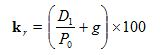(ii)               k = k (1-p)

Here,

D= Expected Dividend per share at the end of year

= Market price of share

g = Growth rate

p= Present tax rate

k= Cost of retained earnings

k= Cost of equity

Problem 1: Current market price of a share of common stock is tk. 120. Expected dividend per share is tk. 20 and it would rise by 5% per share. Calculate cost of retained earnings.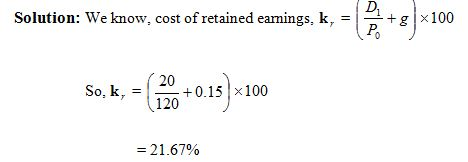Problem 2: The cost of equity share capital is 15% and present tax rate is 40%. Determine cost of retained earnings.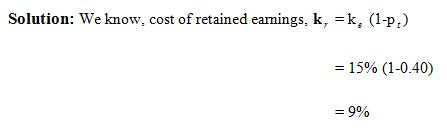5.5 Weighted Average Cost of Capital

The Weighted Average Cost of Capital (WACC) is used in finance to measure a firm’s cost of capital.

The total capital for a firm is the value of its equity (for a firm without outstanding warrents and options, this is the same as the company’s market capitalization) plus the cost of its debt (the cost of debt should be continually updated as the cost of debt changes as a result of interest rate changes). Notice that the “equity” in the debt to equity ratio is the market value of all equity, not the shareholders’ equity on the balance sheet. To calculate the firm’s weighted cost of capital, we must first calculate the costs of the individual financing sources: Cost of Debt, Cost of Preference Capital and Cost of Equity Capital.

Problem:

(a) A firm’s after tax cost of capital of the specific sources are as follows:

Cost of debt                                     8 percent

Cost of preference share                  14 percent

Cost of equity fund                         17 percent

(b) The following is the capital structure

 Sources Amount Debt Tk. 300000 Preference capital Tk. 200000 Equity capital Tk. 500000

(c) Calculate the weighted average cost of capital k.

Solution:

 Sources of funds Amount Proportion Cost (%) Weighted cost (3×4) (1) (2) (3) (4) (5) Debt Tk. 300000 0.3     (30) 0.08 0.024 Preference capital Tk. 200000 0.2     (20) 0.14 0.028 Equity capital Tk. 500000 0.5     (50) 0.17 0.085 Total                          Tk. 1000000            1.00  (100)                                    0.137

So, the weighted average cost of capital k= 13.7 Percent